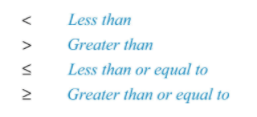# Sitting on the Fence

## OBJECTIVE: To learn how to solve absolute value inequalities

In previous lessons, you learned about inequalities and how to graph them on the real number line. Then you learned about the concept of absolute value and how to solve absolute value equations. Talking About Inequalities: https://www.geogebra.org/m/nrpqkpch Going the Distance: https://www.geogebra.org/m/fyuu6q6n Wearing a Smile or a Frown: https://www.geogebra.org/m/cczmeqjs In this lesson, you're going to learn how to solve absolute value inequalities. As you have learned previously, there are four variations of inequalities:The first applet below illustrates two variations: less than ( < ) and less than or equal to ( ≤ ). The second applet deals with the two other variations: greater than ( > ) and greater than or equal to ( ≥ ). Interact with the applets for a few minutes. In both applets, feel free to move the BIG PURPLE POINTS wherever you'd like.

## HOW TO SOLVE ABSOLUTE VALUE INEQUALITIES

Step 1. Change the absolute value inequality into an absolute value equation. Step 2. Isolate the absolute value expression. Make sure the number on the other side of the equation is positive. If so, continue to Step 3. Other cases will be discussed separately. Step 3. Find the left-hand boundary by equating the algebraic equation inside the absolute value symbol to the negative ( − ) of the number on the other side of the equation and solving for x. Step 4. Find the right-hand boundary by equating the algebraic equation inside the absolute value symbol to the positive ( + ) of the number on the other side of the equation and solving for x. Step 5. The solution set consists of all values of x that are either inside (or equal to) the boundaries for LESS THAN INEQUALITIES ( first applet) or outside (or equal to) to the boundaries for GREATER THAN INEQUALITIES (second applet). "Equal to" applies only when an equal sign accompanies the inequality symbols. These are shown in the applets as solid line boundaries.

## TRY IT YOURSELF ...

Solve the following problems first on a separate sheet of paper, then enter your answers in the corresponding boxes. Use the sliders to change the options. A big "Correct!!!" sign will appear if all your entries and settings are correct. Otherwise, you'll have to redo the problem. Repeat as many times as needed to master the concept.

## In this lesson, you learned how to solve absolute value inequalities.

In future lessons, you'll learn more about real numbers and operations on real numbers. Hope you ENJOYED today's lesson!